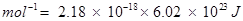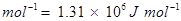### Classification of Elements - Solutions

CBSE Class 11 Chemistry

NCERT Solutions
Chapter 3
Classification of elements and periodicity in Properties

1. What is the basic theme of organisation in the periodic table?

Ans. The basic theme of organisation of elements in the periodic table is to classify the elements in periods and groups according to their properties. This arrangement makes the study of elements and their compounds simple and systematic. In the periodic table, elements with similar properties are placed in the same group.

2. Which important property did Mendeleev use to classify the elements in his periodic table and did he stick to that?

Ans. Mendeleev arranged the elements in his periodic table ordered by atomic weight or mass. He arranged the elements in periods and groups in order of their increasing atomic weight. He placed the elements with similar properties in the same group.

However, he did not stick to this arrangement for long. He found out that if the elements were arranged strictly in order of their increasing atomic weights, then some elements did not fit within this scheme of classification.

Therefore, he ignored the order of atomic weights in some cases. For example, the atomic weight of iodine is lower than that of tellurium. Still Mendeleev placed tellurium (in Group VI) before iodine (in Group VII) simply because iodine's properties are so similar to fluorine, chlorine, and bromine.

3. What is the basic difference in approach between the Mendeleev's Periodic Law and the Modern Periodic Law?

Ans. Mendeleev's Periodic Law states that the physical and chemical properties of elements are periodic functions of their atomic weights. On the other hand, the Modern periodic Law states that the physical and chemical properties of elements are periodic functions of their atomic numbers.

4. On the basis of quantum numbers, justify that the sixth period of the periodic table should have 32 elements.

Ans. In the periodic table of the elements, a period indicates the value of the principal quantum number (n) for the outermost shells. Each period begins with the filling of principal quantum number (n). The value of n for the sixth period is 6. For n = 6, azimuthal quantum number (l) can have values of 0, 1, 2, 3, 4.

According to Aufbau's principle, electrons are added to different orbitals in order of their increasing energies. The energy of the 6d subshell is even higher than that of the 7s subshell.

In the 6th period, electrons can be filled in only 6s, 4f, 5d, and 6 p subshells. Now, 6s has one orbital, 4fhas seven orbitals, 5d has five orbitals, and 6p has three orbitals. Therefore, there are a total of sixteen (1 + 7 + 5 + 3 = 16) orbitals available. According to Pauli's exclusion principle, each orbital can accommodate a maximum of 2 electrons. Thus, 16 orbitals can accommodate a maximum of 32 electrons.

Hence, the sixth period of the periodic table should have 32 elements.

5. In terms of period and group where would you locate the element with Z =114?

Ans. Elements with atomic numbers from Z = 87 to Z = 114 are present in the 7th period of the periodic table. Thus, the element with Z = 114 is present in the 7th period of the periodic table.

In the 7th period, first two elements with Z = 87 and Z= 88 are s-block elements, the next 14 elements excluding Z = 89 i.e., those with Z = 90 - 103 are f - block elements, ten elements with Z = 89 and Z = 104 - 112 are d - block elements, and the elements with Z = 113 - 118 are p - block elements. Therefore, the element with Z = 114 is the second p - block element in the 7th period. Thus, the element with Z= 114 is present in the 7th period and 14th group of the periodic table.

6. Write the atomic number of the element present in the third period and seventeenth group of the periodic table.

Ans. There are two elements in the 1st period and eight elements in the 2nd period., The third period starts with the element with Z = 11. Now, there are eight elements in the third period. Thus, the 3rd period ends with the element with Z = 18 i.e., the element in the 18th group of the third period has Z = 18. Hence, the element in the 17th group of the third period has atomic number Z = 17.

7. Which element do you think would have been named by

(i) Lawrence Berkeley Laboratory

(ii) Seaborg's group?

Ans. (i) Lawrencium (Lr) with Z = 103 and Berkelium (Bk) with Z = 97

(ii) Seaborgium (Sg) with Z = 106

8. Why do elements in the same group have similar physical and chemical properties?

Ans. The physical and chemical properties of elements depend on the number of valence electrons. Elements present in the same group have the same number of valence electrons. Therefore, elements present in the same group have similar physical and chemical properties.

9. What does atomic radius and ionic radius really mean to you?

Ans. Atomic radius is the radius of an atom. It measures the size of an atom. If the element is a metal, then the atomic radius refers to the metallic radius, and if the element is a non-metal, then it refers to the covalent radius. Metallic radius is calculated as half the internuclear distance separating the metal cores in the metallic crystal. For example, the internuclear distance between two adjacent copper atoms in solid copper is 256 pm. Thus, the metallic radius of copper is taken as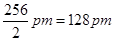.

Covalent radius is measured as the distance between two atoms when they are found together by a single bond in a covalent molecule. For example, the distance between two chlorine atoms in chlorine molecule is 198 pm. Thus, the covalent radius of chlorine is taken as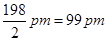.

Ionic radius means the radius of an ion (cation or anion). The ionic radii can be calculated by measuring the distances between the cations and anions in ionic crystals.

Since a cation is formed by removing an electron from an atom, the cation has fewer electrons than the parent atom resulting in an increase in the effective nuclear charge. Thus, a cation is smaller than the parent atom. For example, the ionic radius of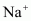ion is 95 pm, whereas the atomic radius of Na atom is 186 pm. On the other hand, an anion is larger in size than its parent atom. This is because an anion has the same nuclear charge, but more electrons than the parent atom resulting in an increased repulsion among the electrons and a decrease in the effective nuclear charge. For example, the ionic radius of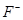ion is 136 pm, whereas the atomic radius of F atom is 64 pm.

10. How does atomic radius vary in a period and in a group? How do you explain the variation?

Ans. Atomic radius generally decreases from left to right across a period. This is because within a period, the outer electrons are present in the same valence shell and the atomic number increases from left to right across a period, resulting in an increased effective nuclear charge. As a result, the attraction of electrons to the nucleus increases.

On the other hand, the atomic radius generally increases down a group. This is because down a group, the principal quantum number (n) increases which results in an increase of the distance between the nucleus and valence electrons.

11. What do you understand by isoelectronic species? Name a species that will be

isoelectronic with each of the following atoms or ions.

(i)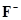(ii) Ar

(iii)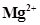(iv) Rb+
Ans. Atoms and ions having the same number of electrons are called isoelectronic species.

(i)ion has 9 + 1 = 10 electrons. Thus, the species isoelectronic with it will also have 10 electrons. Some of its isoelectronic species are Na+ ion (11 - 1 = 10 electrons), Ne (10 electrons), O2- ion (8 + 2 = 10 electrons), and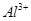ion (13 - 3 = 10 electrons).

(ii) Ar has 18 electrons. Thus, the species isoelectronic with it will also have 18 electrons. Some of its isoelectronic species are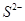ion (16 + 2 = 18 electrons),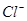ion (17 + 1 = 18 electrons), K+ ion (19 - 1 = 18 electrons), and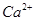ion (20 - 2 = 18 electrons).

(iii)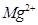ion has 12 - 2 = 10 electrons. Thus, the species isoelectronic with it will also have 10 electrons. Some of its isoelectronic species areion (9 + 1 = 10 electrons), Ne (10 electrons), O2- ion (8 + 2 = 10 electrons), andion (13 - 3 = 10 electrons).

(iv)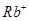ion has 37 - 1 = 36 electrons. Thus, the species isoelectronic with it will also have 36 electrons. Some of its isoelectronic species are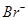ion (35 + 1 = 36 electrons), Kr (36 electrons), and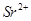ion (38 - 2 = 36 electrons).

12. Consider the following species: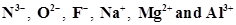(a) What is common in them?

(b) Arrange them in the order of increasing ionic radii.

Ans. (a) Each of the given species (ions) has the same number of electrons (10 electrons). Hence, the given species are isoelectronic.

(b) The ionic radii of isoelectronic species increases with a decrease in the magnitudes of nuclear charge.

The arrangement of the given species in order of their increasing nuclear charge is as follows: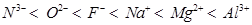Nuclear charge = +7 +8 +9 +11 +12 +13

Therefore, the arrangement of the given species in order of their increasing ionic radii is as follows: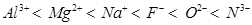13. Explain why cations are smaller and anions larger in radii than their parent atoms?

Ans. A cation has a fewer number of electrons than its parent atom, while its nuclear charge remains the same. As a result, the attraction of electrons to the nucleus is more in a cation than in its parent atom. Therefore, a cation is smaller in size than its parent atom.

On the other hand, an anion has one or more electrons than its parent atom, resulting in an increased repulsion among the electrons and a decrease in the effective nuclear charge. As a result, the distance between the valence electrons and the nucleus is more in anions than in it's the parent atom. Hence, an anion is larger in radius than its parent atom.

14. What is the significance of the terms - 'isolated gaseous atom' and 'ground state' while defining the ionization enthalpy and electron gain enthalpy?

Hint: Requirements for comparison purposes.

Ans. Ionization enthalpy is the energy required to remove an electron from an isolated gaseous atom in its ground state. Although the atoms are widely separated in the gaseous state, there are some amounts of attractive forces among the atoms. To determine the ionization enthalpy, it is impossible to isolate a single atom. But, the force of attraction can be further reduced by lowering the pressure. For this reason, the term 'isolated gaseous atom' is used in the definition of ionization enthalpy.

Ground state of an atom refers to the most stable state of an atom. If an isolated gaseous atom is in its ground state, then less amount energy would be required to remove an electron from it. Therefore, for comparison purposes, ionization enthalpy and electron gain enthalpy must be determined for an 'isolated gaseous atom' and its 'ground state'.

15. Energy of an electron in the ground state of the hydrogen atom is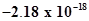J. Calculate the ionization enthalpy of atomic hydrogen in terms of J mol-1.

Ans. It is given that the energy of an electron in the ground state of the hydrogen atom is.

Therefore, the energy required to remove that electron from the ground state of hydrogen atom is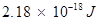.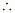Ionization enthalpy of atomic hydrogen =Hence, ionization enthalpy of atomic hydrogen in terms of J